# 手把手教你用Javascript制作随机星星效果图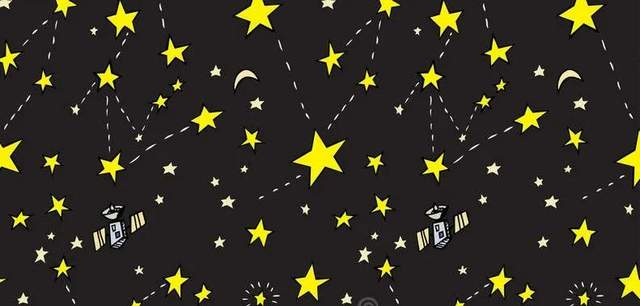# 1. 创建canvas画布

``````<body>
<canvas id='canvas'></canvas>
</body>``````

# 2. 添加css样式。

``````<style type="text/css">
canvas{
border:2px solid #f00;
}
</style>``````

# 3.1 设置canvas画布大小 ，定义需要变量。

``````<script type="text/javascript">
var _canvas=document.getElementById("canvas")
_canvas.width=500;
_canvas.height=500;
var r,g ,b,a;
</script>``````

# 3.2 产生随机圆。

``````for (var j = 0; j < 150; j++) {
arc.x=Math.floor(Math.random()*_canvas.width);
arc.y=Math.floor(Math.random()*_canvas.height);
arc.r=Math.floor(Math.random()*31+10);
r=Math.ceil(Math.random()*256);
g=Math.ceil(Math.random()*256);
b=Math.ceil(Math.random()*256);
a=Math.random();

darw();
}``````

# 3.3 定义draw()方法，通过画星星公式，将圆形转换成星星状 for 循环产生随机位置星星。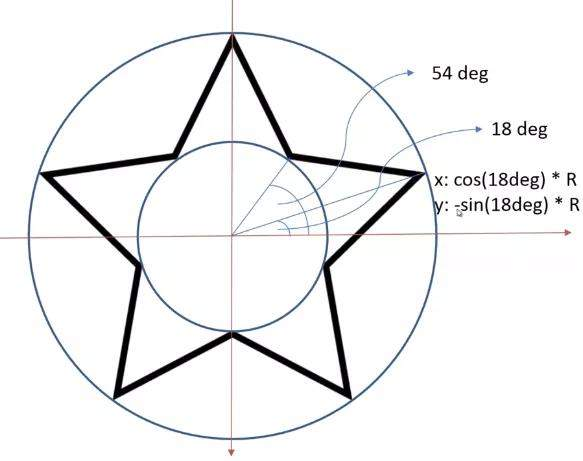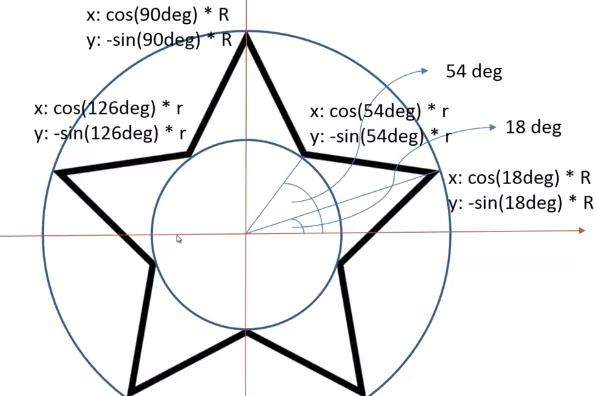``````/* 随机产生星星*/
for (var i = 0; i < 5; i++) {

_ctx.lineTo(Math.cos((18+72*i)/180*Math.PI)*arc.r+arc.x, -Math.sin((18+72*i)/180*Math.PI)*arc.r+arc.y);

_ctx.lineTo(Math.cos((54+72*i)/180*Math.PI)*arc.r/2+arc.x, -Math.sin((54+72*i)/180*Math.PI)*arc.r/2+arc.y);

}``````

# 3.4 随机产生颜色。

Math函数随机产生0-225的RGB值。

``````/* 随机颜色*/
_ctx.fillStyle="rgba(" + r + "," + g + "," + b + "," + a + ")";
_ctx.fill();
_ctx.strokeStyle="rgba(" + r + "," + g + "," + b + "," + a + ")";
_ctx.stroke();
}``````

# 3.5. 调用draw()方法实现功能。

``darw();``

# 五、效果展示

1、点击f12运行到浏览器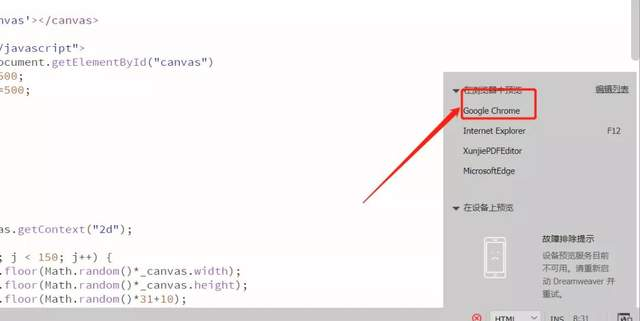2、每次刷新网页，随机产生不一样的星星和随机颜色。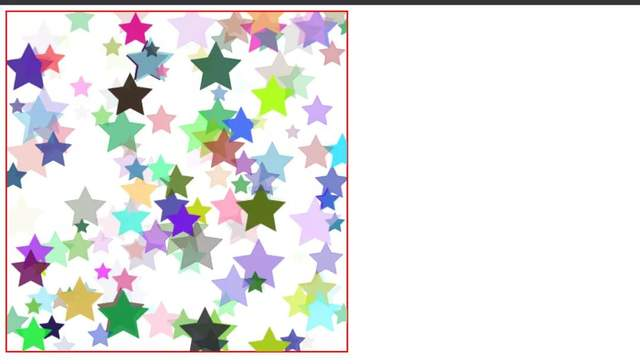# 六、总结

1. 本项目利用canvas画布，实现星星图的效果，以及在运用javascript产生星星效果时，遇到的一些难点进行了分析及提供解决方案。
2. 欢迎大家积极尝试，有时候看到别人实现起来很简单，但是到自己动手实现的时候，总会有各种各样的问题，切勿眼高手低，勤动手，才可以理解的更加深刻。
3. 代码很简单，希望对你有所启发。

IT共享之家——————- End ——————